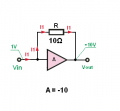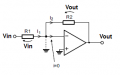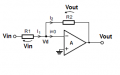# Input impedance of shunt feedback inverting amplifier

#### ray242

Joined Nov 27, 2016
44
Hi, I've been studying negative feedback and I came across this circuit. How can I calculate the input impedance of it? I tried Kirchhoff's law but it gave me equations too long to be solved.

#### Jony130

Joined Feb 17, 2009
5,243

At the beginning we have a ideal (inverting) voltage amplifier with gain equal to A = -10V/V

Next we connect a resistor R = 10Ω between the input and the output of the amplifier.Now let us try to find a input resistance.

Rin = Vin/Iin

Iin = (Vin - Vout)/R = (Vin - A*Vin)/R = Vin * (1 - A)/R

Rin = Vin/Iin = R/(1 - A)

Iin = (1V - (-10V))/10Ω = 1.1A

Rin = 1V/1.1A = 0.909Ω

So, as you can see our Rin resistance is (1 - A) smaller than R if we have inverting amplifier .
And this is what we call a Miller effect.
Also for Inverting amplifier only we can write Rin = R/(1+|A|) where |A| is the absolute value of a gain.

And now if you add a R1 resistor in series with amplifier input Rin will become

Rin = R1 + R/(1+A) and if A is large we can writer Rin ≈ R1.

•ray242

#### ray242

Joined Nov 27, 2016
44

At the beginning we have a ideal (inverting) voltage amplifier with gain equal to A = -10V/V

Next we connect a resistor R = 10Ω between the input and the output of the amplifier.

View attachment 117775

Now let us try to find a input resistance.

Rin = Vin/Iin

Iin = (Vin - Vout)/R = (Vin - A*Vin)/R = Vin * (1 - A)/R

Rin = Vin/Iin = R/(1 - A)

Iin = (1V - (-10V))/10Ω = 1.1A

Rin = 1V/1.1A = 0.909Ω

So, as you can see our Rin resistance is (1 - A) smaller than R if we have inverting amplifier .
And this is what we call a Miller effect.
Also for Inverting amplifier only we can write Rin = R/(1+|A|) where |A| is the absolute value of a gain.

And now if you add a R1 resistor in series with amplifier input Rin will become

Rin = R1 + R/(1+A) and if A is large we can writer Rin ≈ R1.
Thanks Jony!

I actually calculated the input impedance without the series resistor R1. After adding R1 I didn't consider them as two resistor in series but tried to use Kirchhoff's law....and that was really a mess.

Another question, how do we calculate the gain?

#### drc_567

Joined Dec 29, 2008
1,154

#### Jony130

Joined Feb 17, 2009
5,243
I actually calculated the input impedance without the series resistor R1. After adding R1 I didn't consider them as two resistor in series but tried to use Kirchhoff's law....and that was really a mess.
That is not surprise for me. But what is more important here do you understand the final result by using your own intuition without the maths?
Just a simple concept of a Miller effect and R1 series resistance. Or maybe you need a mathematical proof?

Another question, how do we calculate the gain?
If the gain A --->∞Because of a negative feedback the right side of a R1 resistor is virtually shorted (R2/(1+A)) so:

I1 = Vin/R1 and I1 = I2

Vout = -I2*R2 = - Vin/R1 * R2

Vout/Vin= - R2/R1 (closed loop gain)

But with finite gain A (open loop gain) we ca try to use a superposition:Vd' = Vi*(R2/(R1 + R2)

Vd'' = Vo*(R1/(R1 + R2))

Therefore

Vd = Vd' + Vd'' = Vi*(R2/(R1 + R2) + Vo*(R1/(R1 + R2))

We also know that Vd = -Vo/A

-Vo/A = Vi*(R2/(R1 + R2)) + Vo*(R1/(R1 + R2))

And we can solve for Vo/Vin = - (A*R2)/(R1 + A*R1 + R2)

Or we can use KCL and KVL

But we can use another method and find the gain directly.
Let us assume that we now Vo value from that we can find Vd

Vd = - Vo/A

And

I1 = I2 =(Vd - Vo)/R2 = (-Vo/A - Vo)/R2

Vi = I1*R1 +Vo/A = (-Vo/A - Vo)*R1/R2 - Vo/A

$$Vi = \frac{(-\frac{Vo}{A}-Vo)*R1}{R2} - \frac{Vo}{A}$$

Expand the right side

$$Vi = -\frac{Vo}{A}-\frac{VoR1}{R2}-\frac{VoR1}{AR2}$$

Collect in terms of Vo

$$Vi = Vo(-\frac{1}{A}-\frac{R1}{R2}-\frac{R1}{AR2})$$

And finally the closed loop gain

$$\frac{Vo}{Vi}=-\frac{1}{1+\frac{1}{A}(1+\frac{R2}{R1})}*\frac{R2}{R1}$$

Also notice that if we divide

Vin/I1 = Vin/I2 = [(-Vo/A - Vo)*R1/R2 - Vo/A]/[ (-Vo/A - Vo)/R2] we get equation for Rin

Rin = [(-Vo/A - Vo)*R1/R2 - Vo/A]/[ (-Vo/A - Vo)/R2] = (R1 + A R1 + R2)/(1 + A) = R1 + R2/(1+A)

Last edited:
•ray242

#### ray242

Joined Nov 27, 2016
44
That is not surprise for me. But what is more important here do you understand the final result by using your own intuition without the maths?
Just a simple concept of a Miller effect and R1 series resistance. Or maybe you need a mathematical proof?
I think I understand the concept of Miller effect.
Basically the change in input voltage results in a much bigger current to flow through the feedback impedance than it would if the feedback impedance was connected to ground, due to a -A times larger change in output voltage?

Thanks for your reply for the second question. I'll look at it in more detail and see if I can understand it.

#### dannyf

Joined Sep 13, 2015
2,197
assuming the node is the inverting input. from that point forward (towards the opamp), there are two path for the current: one into Ri, and parallel to it into R2. The output voltage, out of phase with the input signal, is -A*Vin. So the current through R2 is (Vin - (-A*Vin)) / R2. or its effective resistance is Vin / (Vin - (-A*Vin)) * R2 = R2 / (1+A).

that resistance is parallel with Ri, giving you total resistance of Ri // R2/(1+A). which is in serial with R1 -> R1 + Ri // R2/(1+A).

•ray242

#### Jony130

Joined Feb 17, 2009
5,243
I think I understand the concept of Miller effect.
Basically the change in input voltage results in a much bigger current to flow through the feedback impedance than it would if the feedback impedance was connected to ground, due to a -A times larger change in output voltage?
It looks like you nailed it.

#### The Electrician

Joined Oct 9, 2007
2,848
assuming the node is the inverting input. from that point forward (towards the opamp), there are two path for the current: one into Ri, and parallel to it into R2. The output voltage, out of phase with the input signal, is -A*Vin. So the current through R2 is (Vin - (-A*Vin)) / R2. or its effective resistance is Vin / (Vin - (-A*Vin)) * R2 = R2 / (1+A).

that resistance is parallel with Ri, giving you total resistance of Ri // R2/(1+A). which is in serial with R1 -> R1 + Ri // R2/(1+A).
The expression "R1 + Ri // R2/(1+A)" is ambiguous. It will evaluate as either "R1 +( Ri // R2)/(1+A)" or "R1 + Ri //( R2/(1+A))", depending on operator precedence.

It's best to include enough parentheses to force the correct evaluation.

•ray242

#### The Electrician

Joined Oct 9, 2007
2,848
Hi, I've been studying negative feedback and I came across this circuit. How can I calculate the input impedance of it? I tried Kirchhoff's law but it gave me equations too long to be solved.

The circuit in your image shows 3 non-idealities, A, Ri and Ro. Do you want to calculate Zin and Vo/Vin with all those non-idealities included?

Jony130 showed calculations that only include a non-ideal A, and dannyf showed Zin including Ri. Exact expressions for the quantities Zin, Zout and Vo/Vin include 3 non-idealities.

#### ray242

Joined Nov 27, 2016
44
The circuit in your image shows 3 non-idealities, A, Ri and Ro. Do you want to calculate Zin and Vo/Vin with all those non-idealities included?

Jony130 showed calculations that only include a non-ideal A, and dannyf showed Zin including Ri. Exact expressions for the quantities Zin, Zout and Vo/Vin include 3 non-idealities.
In my book, although all three non-idealities are drawn, the expression of Zin includes A and Ri, Zout includes A and Ro, gain includes only A. Does it mean sometimes one non-ideality is less important than the others so we can ignore it or do we always have to consider all of them?

Thanks very much!

#### The Electrician

Joined Oct 9, 2007
2,848
It depends.

The non-idealities become more important when A is small, and that happens as the operating frequency increases. And of course, for coursework it's up to the instructor to decide, or the particular application in the real world.

Have you been able to calculate all the non-ideal expressions you needed?

•ray242

#### ray242

Joined Nov 27, 2016
44
It depends.

The non-idealities become more important when A is small, and that happens as the operating frequency increases. And of course, for coursework it's up to the instructor to decide, or the particular application in the real world.

Have you been able to calculate all the non-ideal expressions you needed?
I can now calculate the gain and input impedance but I'm a bit confused about how to calculate the output impedanc. My book says when calculating the output impedance of a series(voltage) feedback amplifier, it's easier to calculate by shorting the input and apply a voltage V at the output and calculate the output current I, then Zout is V/I. I'm not sure I understand why this method is feasible. I tried Zout = open voltage/short current and I got the same answer.

#### MrAl

Joined Jun 17, 2014
8,479
Hi,

If you apply a voltage across a finite resistance you get a finite current, and then the resistance must be:
R=E/I

however when you measure the current 'I' you have to measure through any branches where that current flows.
It works because the output resistance is finite. If it was zero then you'd get an infinite current.

Sometimes the approximations used by another source or individual is not easy to spot as to how they are doing it. Here is appears that for the output impedance they assume the input resistor is infinite, and then later assume that in the denominator since R1 and R2 are so much greater than Zo that in the denominator we can assume Zo is zero, but only in the denominator because only there does it show up as a term not a factor, while in the numerator it shows up as a factor so it cant be eliminated.
To see this you would probably have to do a full analysis of the output mathematically and then compare it to the Zout formula give, then try to figure out what the assumptions are, and they usually involve either making something zero or making something infinite when those changes will not affect the percentage of the result by too much. For a quick fictional example, if we get Zout=1.001 with the input resistor present and we get Zout=1.002 with it absent, it makes sense to leave it out, as long as we can prove this for any set of values that are within reason using typical values with typical ranges for their values (the output impedance Zo is almost always much smaller than the feedback resistors for example).

•ray242

#### The Electrician

Joined Oct 9, 2007
2,848
I can now calculate the gain and input impedance but I'm a bit confused about how to calculate the output impedanc. My book says when calculating the output impedance of a series(voltage) feedback amplifier, it's easier to calculate by shorting the input and apply a voltage V at the output and calculate the output current I, then Zout is V/I. I'm not sure I understand why this method is feasible. I tried Zout = open voltage/short current and I got the same answer.
How did you calculate the input impedance? What result did you get?